• mnist手写数字识别jupyter
千次阅读
2020-07-17 16:51:08

看了《python神经网络编程》，跟着书上敲了一下mnist手写数字的代码，对神经网络有了初步的了解。

此项目为三层神经网络识别，激活函数采用sigmoid函数，数据集为mnist手写数字集，训练集包括60000个样本，测试集10000个样本。

第一部分,创建神将网络模型

import numpy
import matplotlib.pyplot
#%matplotlib inline用在Jupyter notebook中（代替plt.show()）,使用%matplotlib命令可以将matplotlib的图表直接嵌入到Notebook之中
%matplotlib inline
#sigmoid函数，在这组函数中，S函数称为expit（）
import scipy.special

class neuralNetwork:
def __init__(self,inputnodes,hiddennodes,outputnodes,learningrate):
self.inodes = inputnodes  #输入节点
self.hnodes = hiddennodes #隐藏节点
self.onodes = outputnodes #输出节点
self.lr = learningrate    #学习率

#         初始化参数
self.wih=(numpy.random.rand(self.hnodes,self.inodes )-0.5)
self.woh=(numpy.random.rand(self.onodes,self.hnodes)-0.5)

#        激活函数为sigmoid函数，使用lambda创建函数（匿名函数），方便快捷
self.activation_function = lambda x:scipy.special.expit(x)

pass

#     训练d代码
def train(self,input_list,target_list):
#         将输入转换为numpy数组，dmin指定生成数组的最小维度
inputs = numpy.array(input_list,ndmin=2).T
targets = numpy.array(target_list,ndmin=2).T
#     计算隐藏层
hidden_inputs = numpy.dot(self.wih,inputs)
#     调用sigmoid激活函数
hidden_outputs = self.activation_function(hidden_inputs)
#      输出层
final_inputs=numpy.dot(self.woh,hidden_outputs)
final_outputs = self.activation_function(final_inputs)

#         计算误差
error = targets-final_outputs
#     隐藏层误差节点反向传播的误差
hidden_error = numpy.dot(self.woh.T,error)

#       优化权重, 正常对应元素相乘,·乘是矩阵点击
#       transpose（）简单来说，就相当于数学中的转置，在矩阵中，转置就是把行与列相互调换位置；
self.woh += self.lr * numpy.dot((error * final_outputs * (1.0-final_outputs)),numpy.transpose(hidden_outputs))
self.wih += self.lr * numpy.dot((hidden_error*hidden_outputs*(1.0-hidden_outputs)),numpy.transpose(inputs))

pass

#     查询函数
def query(self,inputs):

inputs = numpy.array(inputs,ndmin=2).T

hidden_inputs = numpy.dot(self.wih,inputs)
hidden_outputs = self.activation_function(hidden_inputs)
final_inputs=numpy.dot(self.woh,hidden_outputs)
final_outputs = self.activation_function(final_inputs)

return final_outputs
pass

第二部分 训练网络

inputnodes=28*28
hiddennodes=100
outputnodes=10
learningrate=0.1
# 创造神经网络实例
n = neuralNetwork(inputnodes,hiddennodes,outputnodes,learningrate)

# 获取mnist_train数据集
training_date_file = open("mnist_train.csv","r")
training_date_file.close()

a=0
for record in training_date_list:

# 用“，”分割长的字符串值，拆分为单个值
all_values = record.split(',')
# 第一个值为标签，忽略， 将剩余784个值转换为28*28数组
# numpy.asfarray（）将文本字符串转换为实数并创建这些数字的数组，
# 因为文件是用文本读取的，每一条记录仍然是文本，用“，”分割得到的仍然是文本
# all_values[1:]/255.0*0.99)+0.01 是将输入颜色从0-255缩小到0.01-1.00，最小值为0.01是避免先前观察到的0值输入最终会人为地造成权重更新失败
inputs =( numpy.asfarray(all_values[1:])/255.0*0.99)+0.01

#     将训练目标标签从字符串形式转换为整数形式，正确元素设置为0.99 标签“0”转换为整数0
targets = numpy.zeros(outputnodes)+0.01
targets[int(all_values)]=0.99
n.train(inputs,targets)

#     a用来计数，查看训练进度
a=a+1
if(a%10000==0):
print(a)

pass


网络训练结束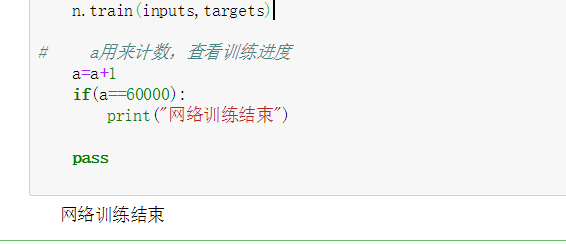第三部分 网络测试

# 测试网络

test_date_file = open("mnist_test.csv","r")
test_date_file.close()

# 创建空列表，用作积分卡，在测试每条记录之后都会进行更新
scorecard=[]
b=0
for record in test_date_list:
all_values = record.split(',')
inputs = (numpy.asfarray(all_values[1:])/255.0*0.99)+0.01
#     目标标签
correct_label=int(all_values)
outputs = n.query(inputs)
#     测试所得标签
label=numpy.argmax(outputs)

#   如果相等，在记分卡追加1,不相等0
if(label==correct_label):
scorecard.append(1)
else:
scorecard.append(0)

#    b用来计数，查看测试进度
b= b+1
if(b%1000==0):
print(b)

pass

#输出正确率
scorecard_array=numpy.asarray(scorecard)
print("Accuracy= ",scorecard_array.sum()/scorecard_array.size)


结果: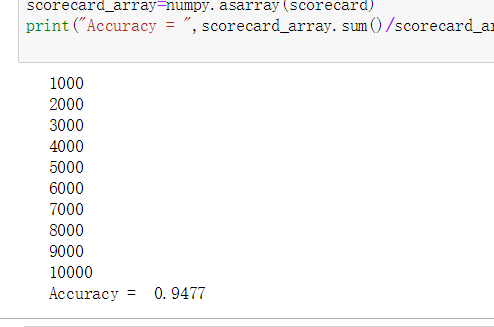模型改进:

1.调整学习率,变大,变小

• 当前学习率为0.1,准确率为0.9477
• 调整学习率为0.2,学习率有所提升.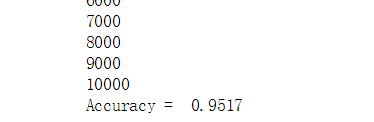• 调整为0.05,学习率有所下降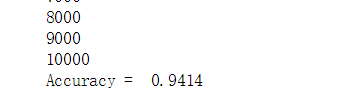2.多次运行,训练一次称为一个世代

3.改变网络形状,改变隐藏节点的数量等

更多相关内容
• MNIST手写数字识别我将在PyTorch中构建一个简单的神经网络，并训练它使用MNIST数据集来识别手写数字。 在MNIST数据集上训练分类器可以被视为图像识别的世界。 创建神经网络的步骤如下： 搭建环境准备数据集建立网络...
• mnist手写数字识别，代码可在正确环境下直接运行。 欢迎下载AI
• 3层神经网络实现手写数字识别（代码+数据 jupyter直接运行看结果） 另有两组数据：处理后Mnist数据集 处理正确率接近97% 适合初学者学习
• MNIST手写数字数据集介绍2. 神经网络程序说明3. 网络结构说明 我的环境： 语言环境：Python3.6.5 编译器：jupyter notebook 深度学习环境：TensorFlow2 一、前期工作 1. 设置GPU（如果使用的是CPU可以忽略这步）...

本文为🔗365天深度学习训练营 内部限免文章（版权归 K同学啊 所有）
参考本文所写记录性文章，请在文章开头注明以下内容，复制粘贴即可

>- **🍨 本文为[🔗365天深度学习训练营](https://mp.weixin.qq.com/s/k-vYaC8l7uxX51WoypLkTw) 内部限免文章（版权归 *K同学啊* 所有）**
>- **🍦 参考文章地址： [🔗深度学习100例-循环神经网络（RNN）心脏病预测 | 第46天](https://blog.csdn.net/qq_38251616/article/details/126345651)**
>- **🍖 作者：[K同学啊 | 接辅导、程序定制](https://mp.weixin.qq.com/s/k-vYaC8l7uxX51WoypLkTw)**


我的环境：

• 语言环境：Python3.6.5
• 编译器：jupyter notebook
• 深度学习环境：TensorFlow2
• 数据和代码：📌【传送门】

来自专栏：【深度学习100例】

# 一、前期工作

## 1. 设置GPU（如果使用的是CPU可以忽略这步）

import tensorflow as tf
gpus = tf.config.list_physical_devices("GPU")

if gpus:
gpu0 = gpus #如果有多个GPU，仅使用第0个GPU
tf.config.experimental.set_memory_growth(gpu0, True) #设置GPU显存用量按需使用
tf.config.set_visible_devices([gpu0],"GPU")


## 2. 导入数据

import tensorflow as tf
from tensorflow.keras import datasets, layers, models
import matplotlib.pyplot as plt

# 导入mnist数据，依次分别为训练集图片、训练集标签、测试集图片、测试集标签
(train_images, train_labels), (test_images, test_labels) = datasets.mnist.load_data()


## 3. 归一化

数据归一化作用

• 使不同量纲的特征处于同一数值量级，减少方差大的特征的影响，使模型更准确
• 加快学习算法的收敛速度

更详解的介绍请参考文章：🔗归一化与标准化

# 将像素的值标准化至0到1的区间内。(对于灰度图片来说，每个像素最大值是255，每个像素最小值是0，也就是直接除以255就可以完成归一化。)
train_images, test_images = train_images / 255.0, test_images / 255.0
# 查看数据维数信息
train_images.shape,test_images.shape,train_labels.shape,test_labels.shape
"""
输出：((60000, 28, 28), (10000, 28, 28), (60000,), (10000,))
"""


## 4. 可视化图片

# 将数据集前20个图片数据可视化显示
# 进行图像大小为20宽、10长的绘图(单位为英寸inch)
plt.figure(figsize=(20,10))
# 遍历MNIST数据集下标数值0~49
for i in range(20):
# 将整个figure分成5行10列，绘制第i+1个子图。
plt.subplot(2,10,i+1)
# 设置不显示x轴刻度
plt.xticks([])
# 设置不显示y轴刻度
plt.yticks([])
# 设置不显示子图网格线
plt.grid(False)
# 图像展示，cmap为颜色图谱，"plt.cm.binary"为matplotlib.cm中的色表
plt.imshow(train_images[i], cmap=plt.cm.binary)
# 设置x轴标签显示为图片对应的数字
plt.xlabel(train_labels[i])
# 显示图片
plt.show()## 5. 调整图片格式

#调整数据到我们需要的格式
train_images = train_images.reshape((60000, 28, 28, 1))
test_images = test_images.reshape((10000, 28, 28, 1))

train_images.shape,test_images.shape,train_labels.shape,test_labels.shape
"""
输出：((60000, 28, 28, 1), (10000, 28, 28, 1), (60000,), (10000,))
"""


# 二、构建CNN网络模型

# 创建并设置卷积神经网络
# 卷积层：通过卷积操作对输入图像进行降维和特征抽取
# 池化层：是一种非线性形式的下采样。主要用于特征降维，压缩数据和参数的数量，减小过拟合，同时提高模型的鲁棒性。
# 全连接层：在经过几个卷积和池化层之后，神经网络中的高级推理通过全连接层来完成。
model = models.Sequential([
# 设置二维卷积层1，设置32个3*3卷积核，activation参数将激活函数设置为ReLu函数，input_shape参数将图层的输入形状设置为(28, 28, 1)
# ReLu函数作为激活励函数可以增强判定函数和整个神经网络的非线性特性，而本身并不会改变卷积层
# 相比其它函数来说，ReLU函数更受青睐，这是因为它可以将神经网络的训练速度提升数倍，而并不会对模型的泛化准确度造成显著影响。
layers.Conv2D(32, (3, 3), activation='relu', input_shape=(28, 28, 1)),
#池化层1，2*2采样
layers.MaxPooling2D((2, 2)),
# 设置二维卷积层2，设置64个3*3卷积核，activation参数将激活函数设置为ReLu函数
layers.Conv2D(64, (3, 3), activation='relu'),
#池化层2，2*2采样
layers.MaxPooling2D((2, 2)),

layers.Flatten(),                    #Flatten层，连接卷积层与全连接层
layers.Dense(64, activation='relu'), #全连接层，特征进一步提取，64为输出空间的维数，activation参数将激活函数设置为ReLu函数
layers.Dense(10)                     #输出层，输出预期结果，10为输出空间的维数
])
# 打印网络结构
model.summary()# 三、编译模型

"""
这里设置优化器、损失函数以及metrics
这三者具体介绍可参考我的博客：
https://blog.csdn.net/qq_38251616/category_10258234.html
"""
# model.compile()方法用于在配置训练方法时，告知训练时用的优化器、损失函数和准确率评测标准
model.compile(
# 设置损失函数为交叉熵损失函数（tf.keras.losses.SparseCategoricalCrossentropy()）
# from_logits为True时，会将y_pred转化为概率（用softmax），否则不进行转换，通常情况下用True结果更稳定
loss=tf.keras.losses.SparseCategoricalCrossentropy(from_logits=True),
# 设置性能指标列表，将在模型训练时监控列表中的指标
metrics=['accuracy'])


# 四、训练模型

"""
这里设置输入训练数据集（图片及标签）、验证数据集（图片及标签）以及迭代次数epochs
关于model.fit()函数的具体介绍可参考我的博客：
https://blog.csdn.net/qq_38251616/category_10258234.html
"""
history = model.fit(
# 输入训练集图片
train_images,
# 输入训练集标签
train_labels,
# 设置10个epoch，每一个epoch都将会把所有的数据输入模型完成一次训练。
epochs=10,
# 设置验证集
validation_data=(test_images, test_labels))# 五、预测

通过下面的网络结构我们可以简单理解为，输入一张图片，将会得到一组数，这组代表这张图片上的数字为0~9中每一个数字的几率，out数字越大可能性越大。在这一步中部分同学会因为 matplotlib 版本原因报 Invalid shape (28, 28, 1) for image data 的错误提示，可以将代码改为 plt.imshow(test_images.reshape(28,28))

plt.imshow(test_images)输出测试集中第一张图片的预测结果

pre = model.predict(test_images) # 对所有测试图片进行预测
pre # 输出第一张图片的预测结果# 六、知识点详解

本文使用的是最简单的CNN模型- -LeNet-5，如果是第一次接触深度学习的话，可以先试着把代码跑通，然后再尝试去理解其中的代码。

## 1. MNIST手写数字数据集介绍

MNIST手写数字数据集来源于是美国国家标准与技术研究所，是著名的公开数据集之一。数据集中的数字图片是由250个不同职业的人纯手写绘制，数据集获取的网址为：http://yann.lecun.com/exdb/mnist/（下载后需解压）。我们一般会采用(train_images, train_labels), (test_images, test_labels) = datasets.mnist.load_data()这行代码直接调用，这样就比较简单

MNIST手写数字数据集中包含了70000张图片，其中60000张为训练数据，10000为测试数据，70000张图片均是28*28，数据集样本如下：如果我们把每一张图片中的像素转换为向量，则得到长度为28*28=784的向量。因此我们可以把训练集看成是一个[60000,784]的张量，第一个维度表示图片的索引，第二个维度表示每张图片中的像素点。而图片里的每个像素点的值介于0-1之间。## 2. 神经网络程序说明

神经网络程序可以简单概括如下：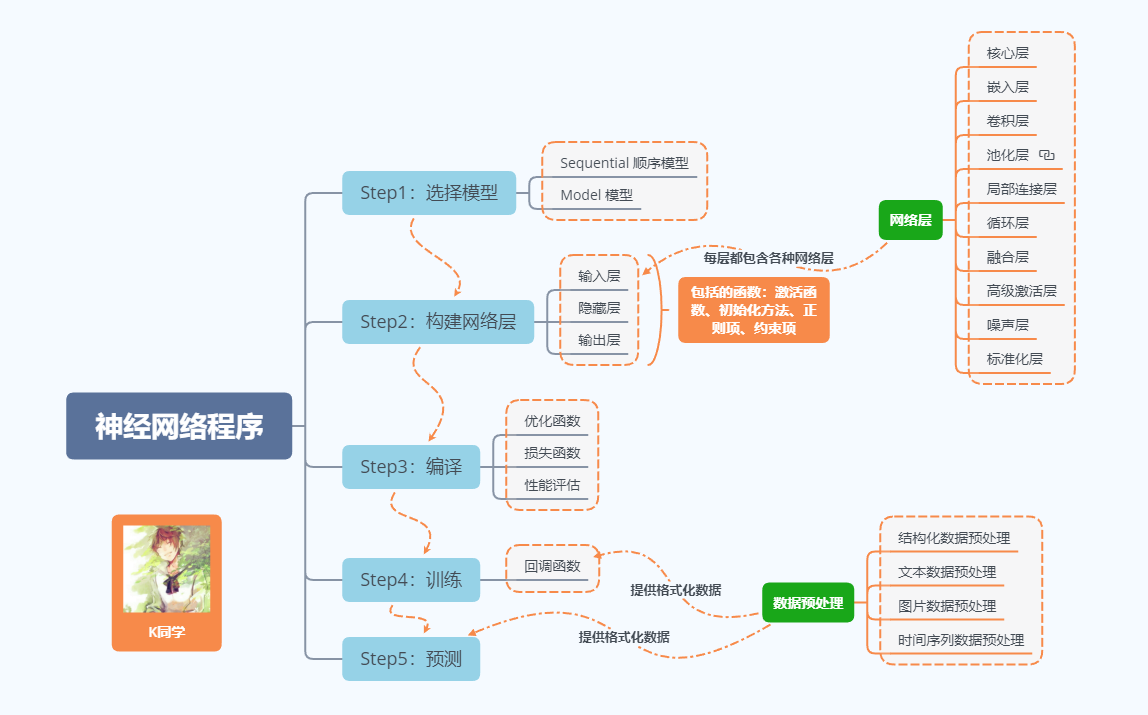## 3. 网络结构说明

模型的结构各层的作用

• 输入层：用于将数据输入到训练网络
• 卷积层：使用卷积核提取图片特征
• 池化层：进行下采样，用更高层的抽象表示图像特征
• Flatten层：将多维的输入一维化，常用在卷积层到全连接层的过渡
• 全连接层：起到“特征提取器”的作用
• 输出层：输出结果

其他精彩内容：

《深度学习100例》专栏直达：【传送门】

• ✨微信众号（K同学啊）后台回复【DL+天数】可获取《深度学习100例》的数据
• ✨微信交流群：加我微信（mtyjkh_）拉你进群，不懂就问，备注：CSDN+来意

我的微信：展开全文minst
• 该资源以jupyter notebook为编辑器，使用 tensorflow为框架，实现了对Mnist手写数据集的识别。压缩包中包括在jupyter notebook中可以打开和运行的源代码MNIST.ipynb，以及Mnist手写数据集的数据MNIST_data。
• tensorflow分类应用（MNIST手写数字识别）的jupyter笔记
• 卷积神经网络（CNN）实现mnist手写数字识别

In

# 设置GPU如果使用的是CPU可以忽略这步

import tensorflow as tf
gpus=tf.config.list_physical_devices("GPU")
if gpus:
gpu0=gpus
tf.config.experimental.set_memory_growth(gpu0,True)
tf.config.set_visible_devices([gpu0],"GPU")


In

# ## 2. 导入数据

import tensorflow as tf
from tensorflow.keras import datasets,layers,models
import matplotlib.pyplot as plt


In

# ## 3. 归一化

train_images,test_images=train_images/255.0,test_images/255.0
train_images.shape,test_images.shape,train_labels.shape,test_labels.shape


In

# ## 4. 可视化

class_names=['airplane','automobile','bird','cat','deer','dog','frog','horse','ship','truck']
plt.figure(figsize=(20,10))
for i in range(20):
plt.subplot(5,10,i+1)
plt.xticks([])
plt.yticks([])
plt.grid(False)
plt.imshow(train_images[i],cmap=plt.cm.binary)
plt.xlabel(class_names[train_labels[i]])
plt.show()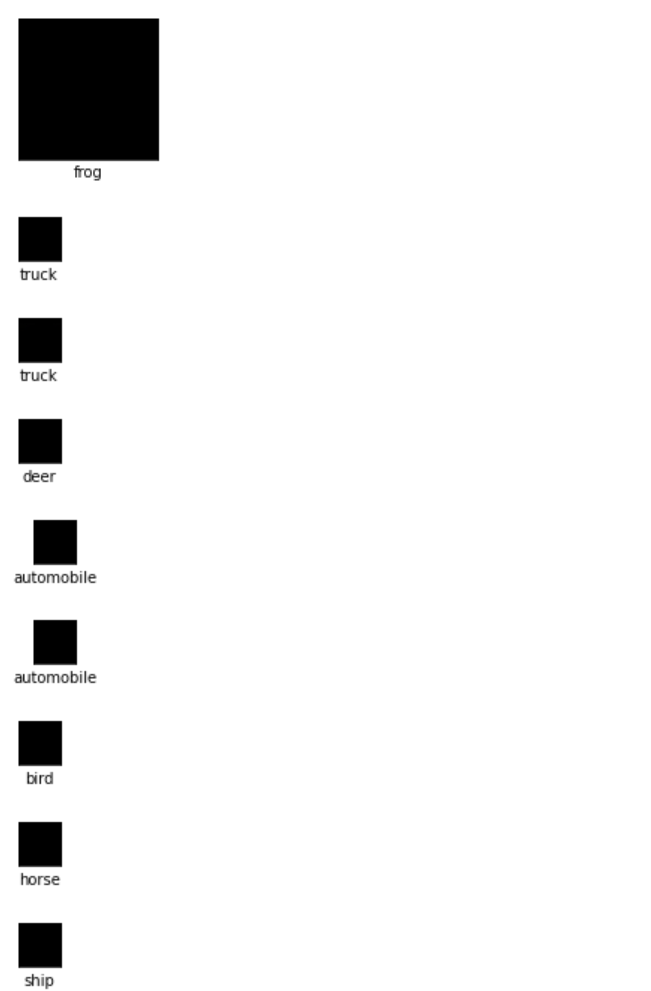In

# # 二、构建CNN网络

model=models.Sequential([
layers.Conv2D(32,(3,3),activation='relu',input_shape=(32,32,3)),
layers.MaxPooling2D((2,2)),
layers.Conv2D(64,(3,3),activation='relu'),
layers.MaxPooling2D((2,2)),
layers.Conv2D(64,(3,3),activation='relu'),
layers.Flatten(),
layers.Dense(64,activation='relu'),
layers.Dense(10)
])
model.summary()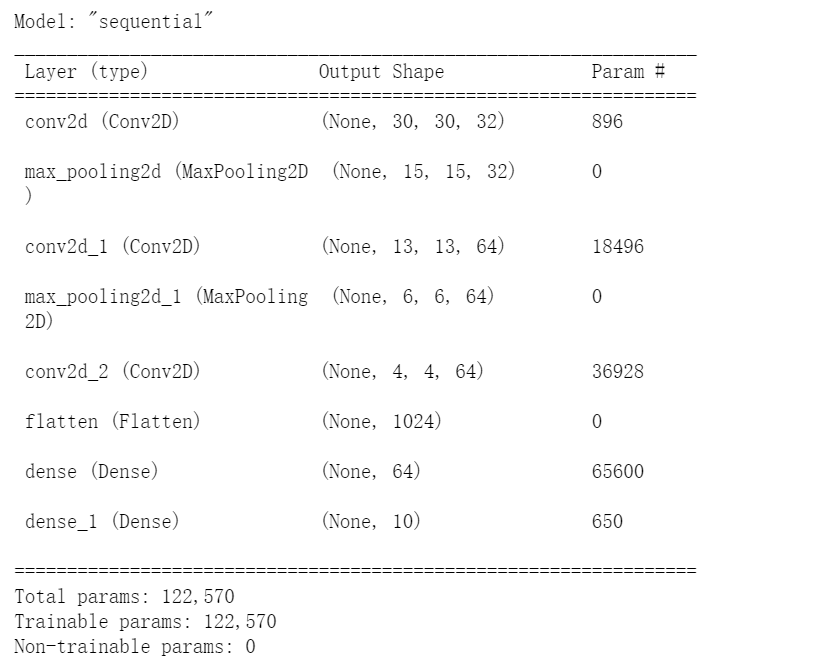In

# # 三、编译

model.compile(optimizer='adam',
loss=tf.keras.losses.SparseCategoricalCrossentropy(from_logits=True),
metrics=['accuracy']
)


In

# # 四、训练模型

history=models.fit(train_images,train_labels,epochs=10,validation_data=(test_images,test_labels))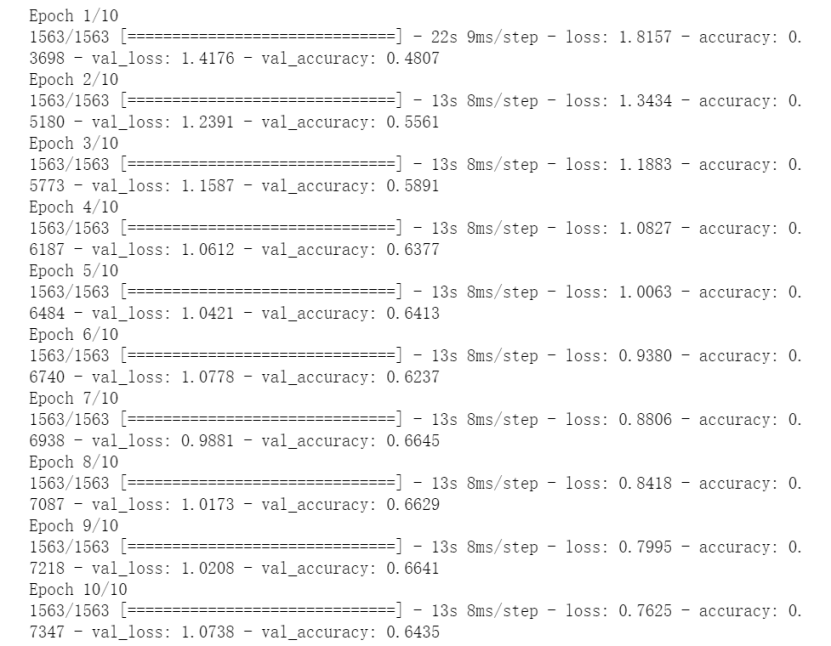In

# # 五、预测

plt.imshow(test_images)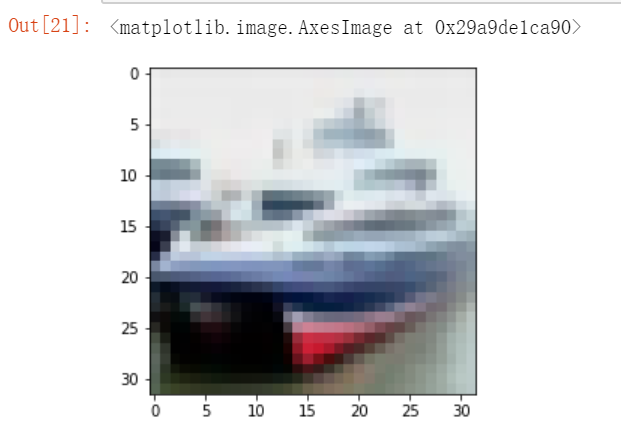In

import numpy as np
pre =model.predict(test_images)
print(class_names[np.argmax(pre)])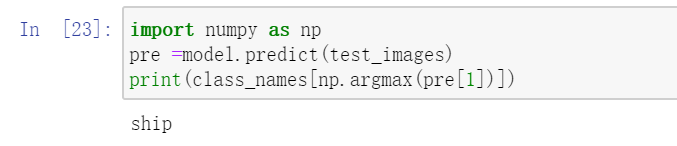In

# # 六、模型评估

import matplotlib.pyplot as plt

plt.plot(history.history['accuracy'], label='accuracy')
plt.plot(history.history['val_accuracy'], label = 'val_accuracy')
plt.xlabel('Epoch')
plt.ylabel('Accuracy')
plt.ylim([0.5, 1])
plt.legend(loc='lower right')
plt.show()

test_loss, test_acc = model.evaluate(test_images,  test_labels, verbose=2)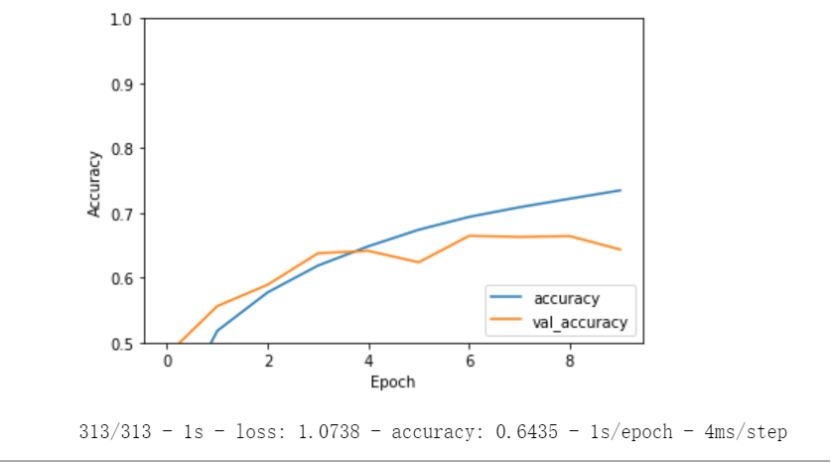In

# 打印精度

print(test_acc)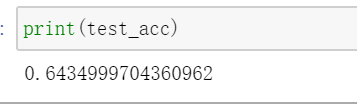展开全文机器学习
• MNIST数据集(MixedNationalInstituteofStandardsandTechnologydatabase)是美国国家标准与技术研究院收集整理的大型手写数字数据库，包含6万个示例的训练集以及1万个示例的测试集。收集时间1998年MNIST数字文字识别...

## 1 介绍一下

MNIST数据集 (Mixed National Institute of Standards and Technology database) 是美国国家标准与技术研究院收集整理的大型手写数字数据库，包含 6万 个示例的训练集以及 1万 个示例的测试集。

收集时间：1998年

MNIST数字文字识别数据集数据量不太多，而且是单色的图像，比较简单，很适合深度学习的初学者用来练习建立模型、训练、预测。

## 2 环境准备

在此之前，我已经装好了Anaconda，并且创建了两个虚拟环境，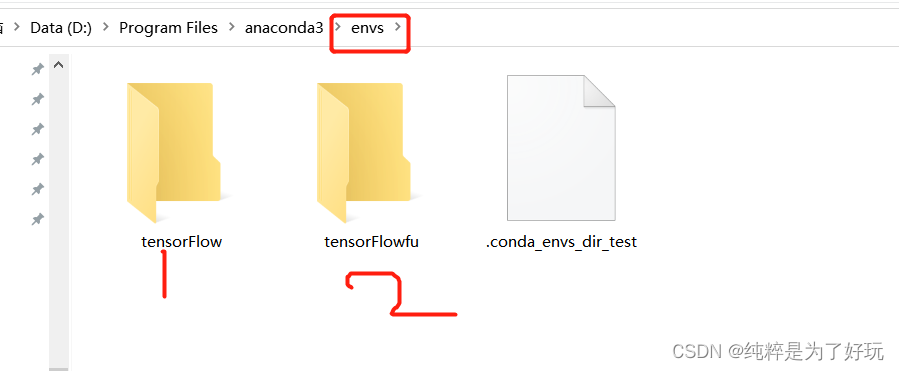其实，之所以用虚拟环境，是为了解决包之间兼容性问题。

下面激活虚拟环境

conda activate tensorFlowfu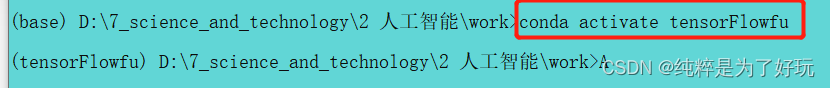启动 jupyter notebook

 jupyter notebook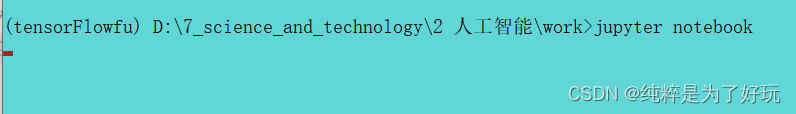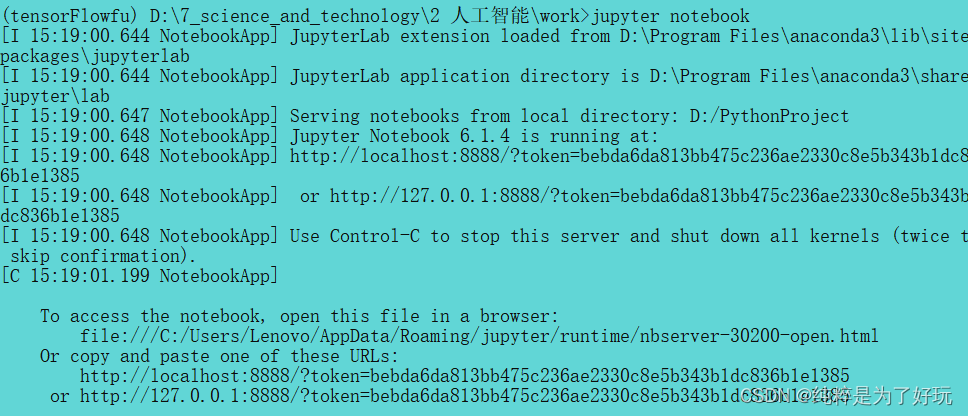## 3 导入数据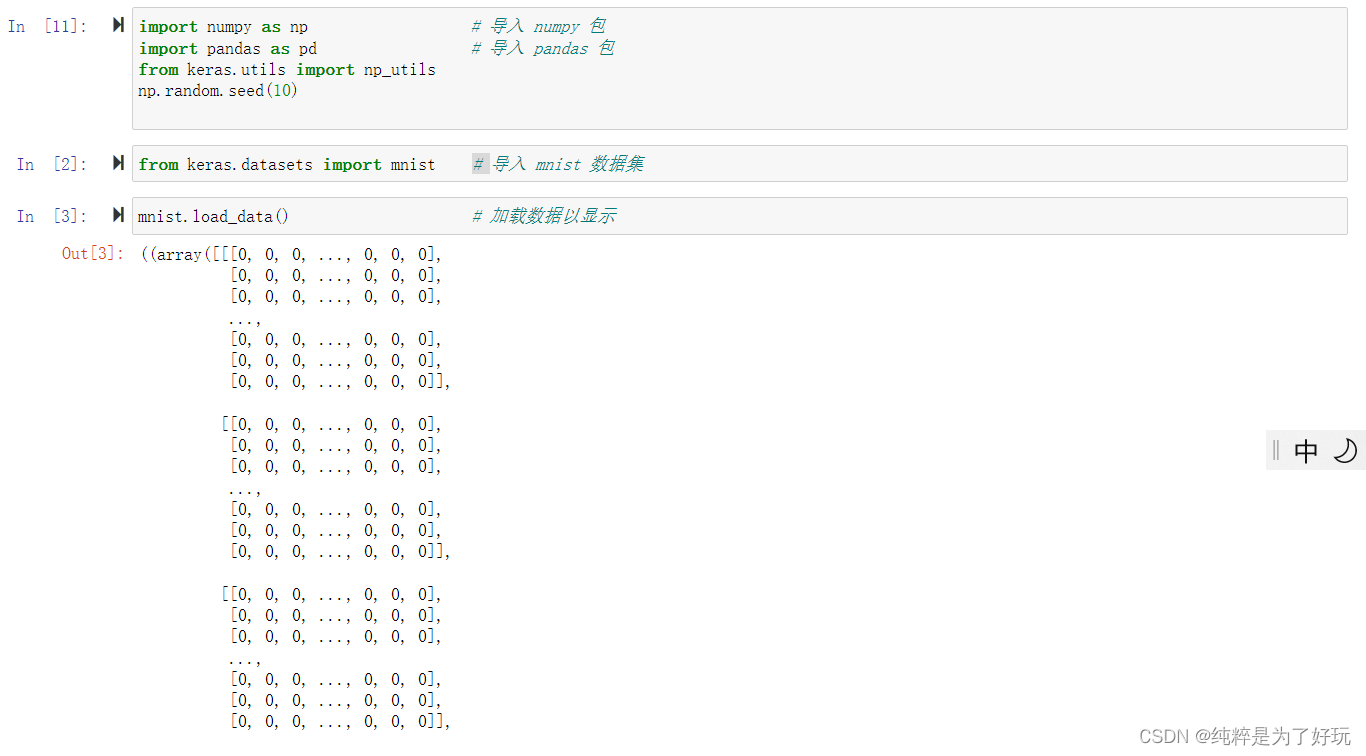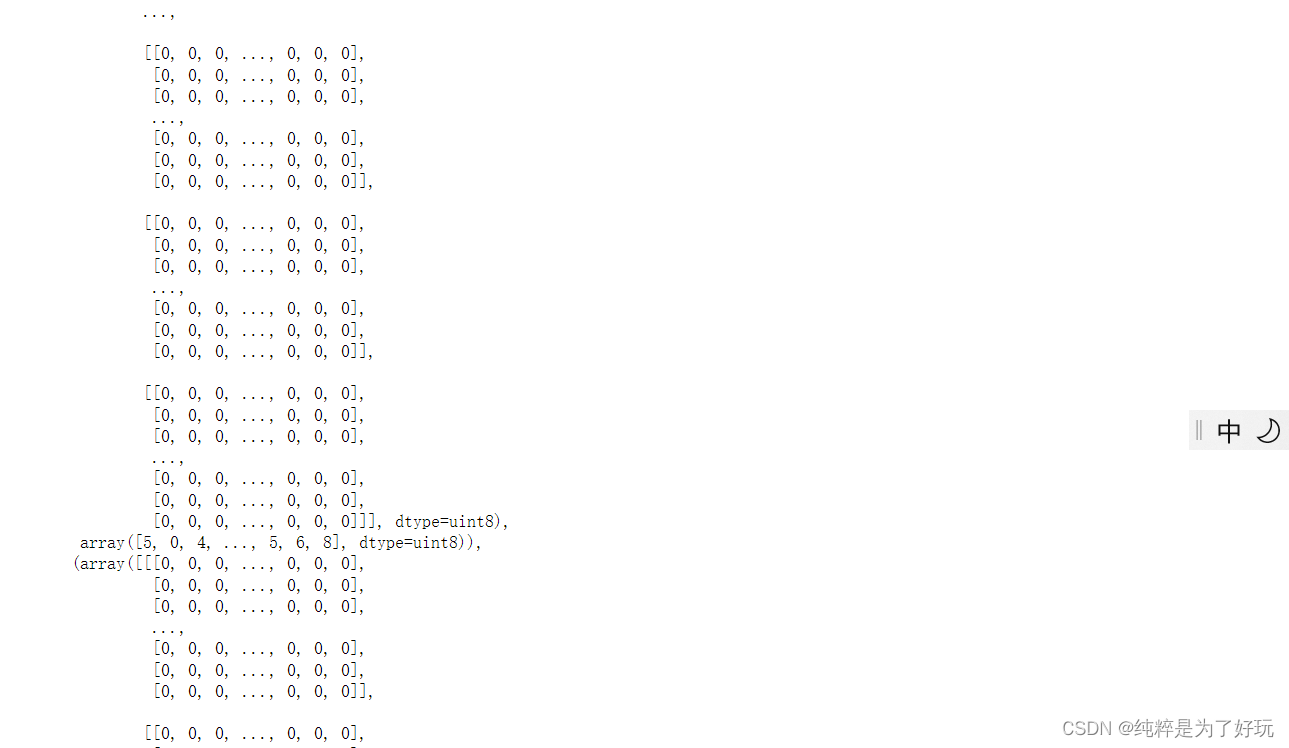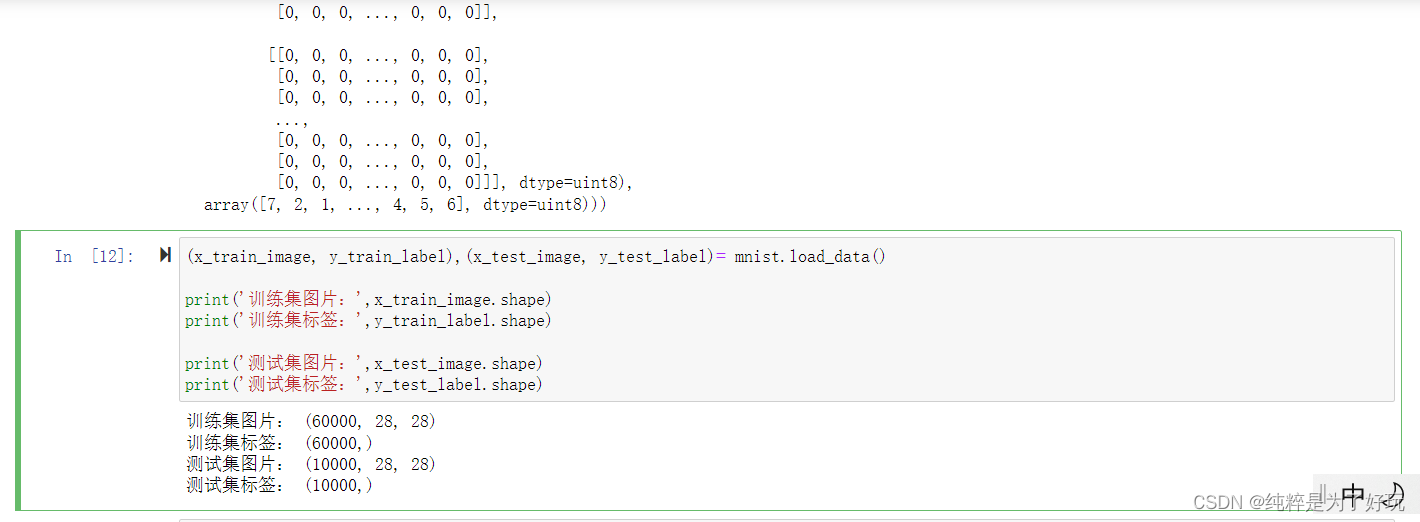MNIST数据集里的每一个图片，其实就是28 * 28的矩阵，一共有六万个矩阵，构成了一个60000 * 28 * 28的数组。

每个矩阵都对应一个标签，也就是一个数值，测试集的标签，是一个 1 * 60000 的数组。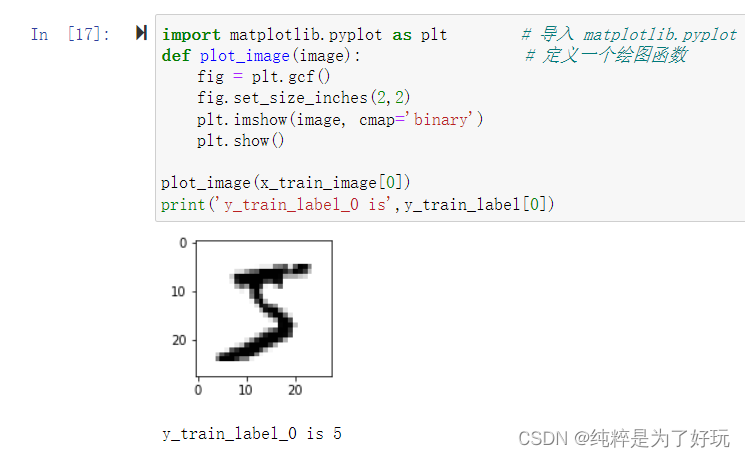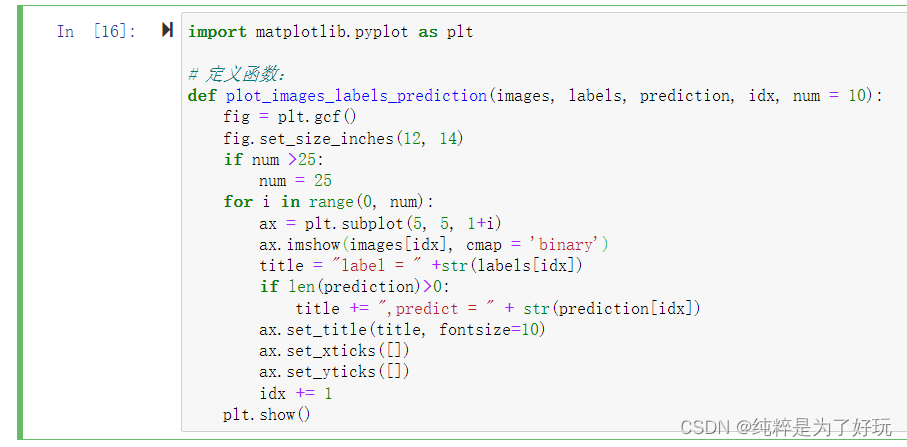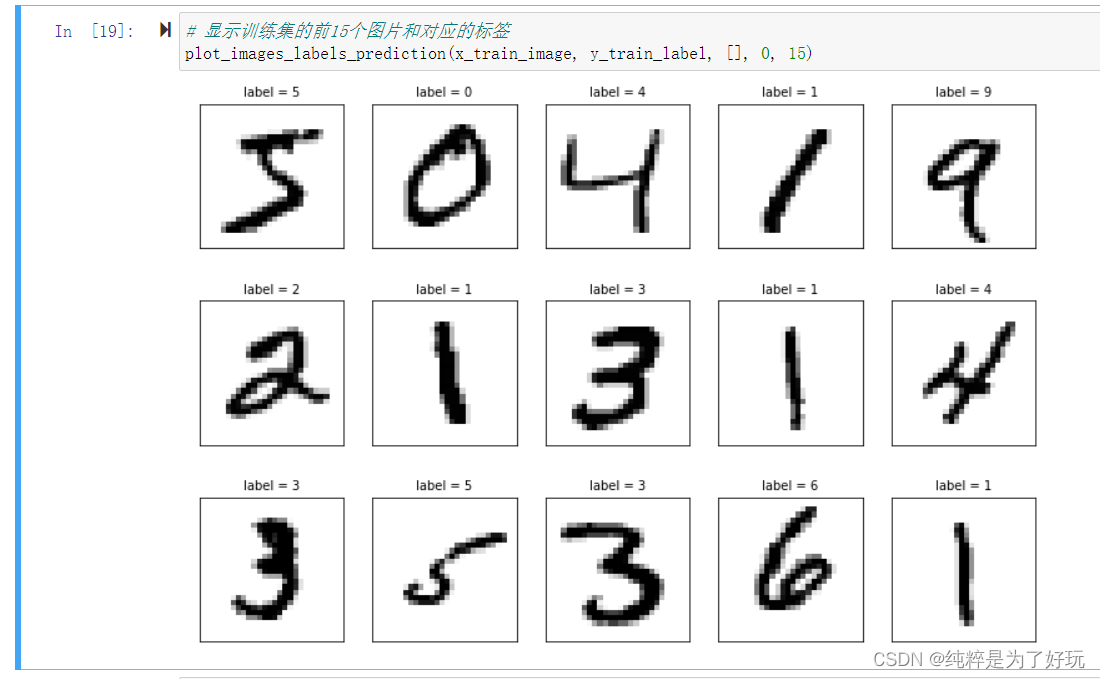## 4 数据预处理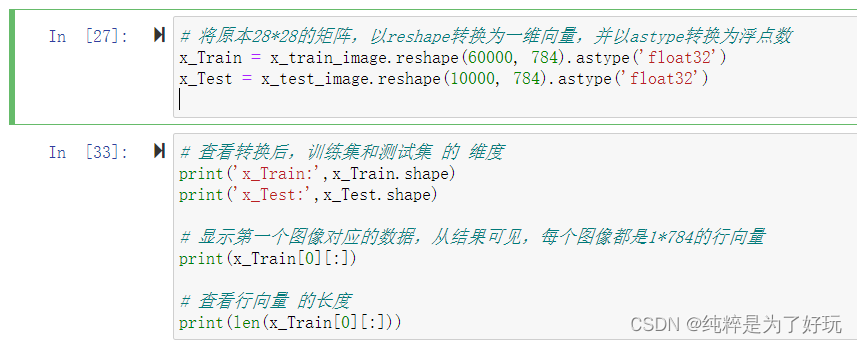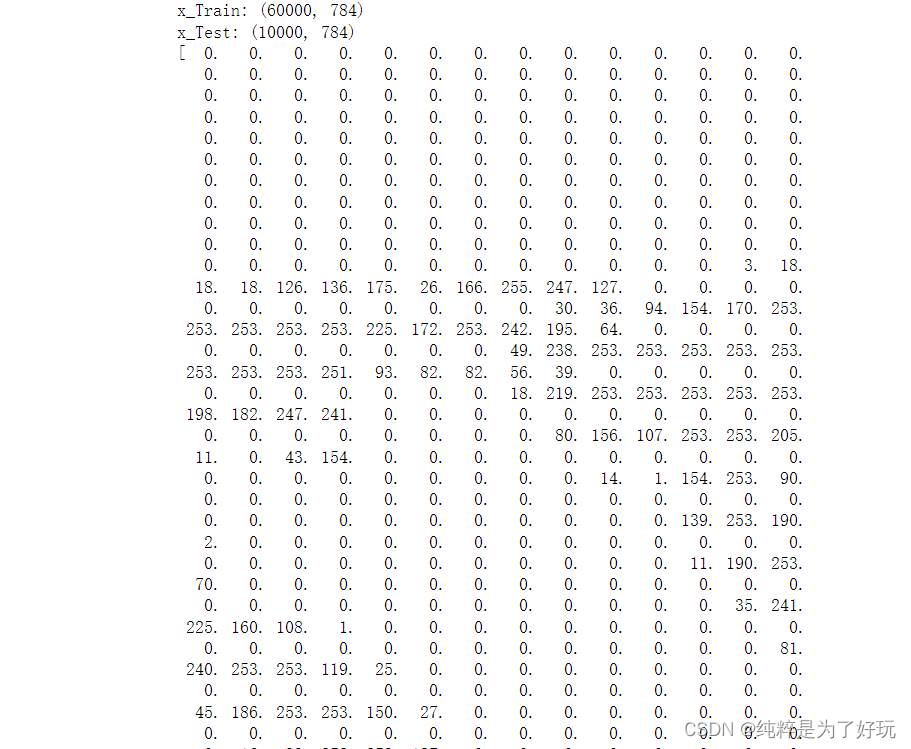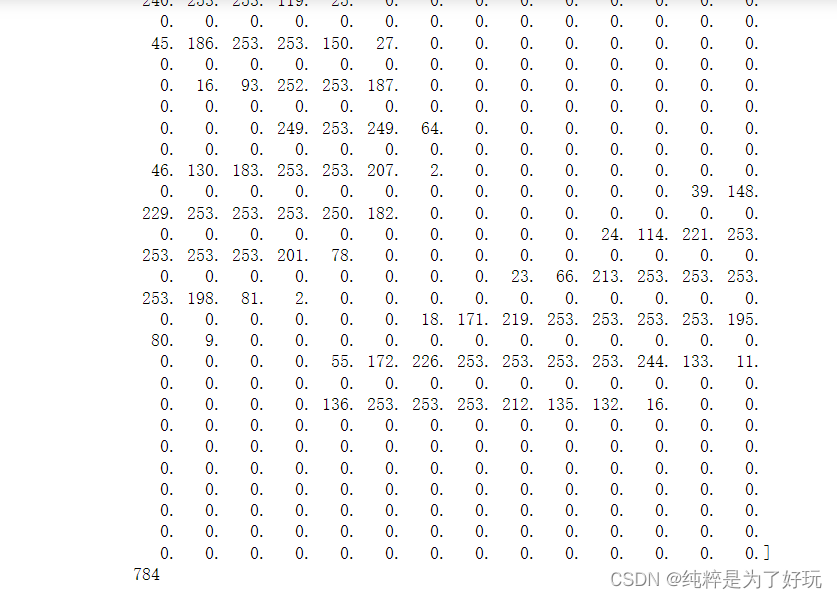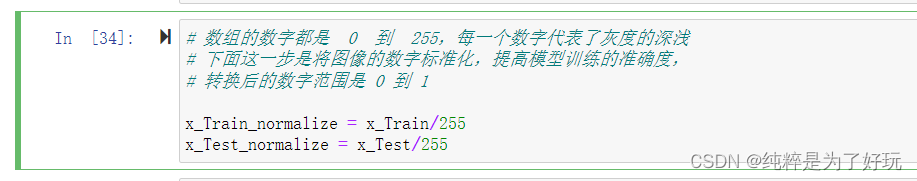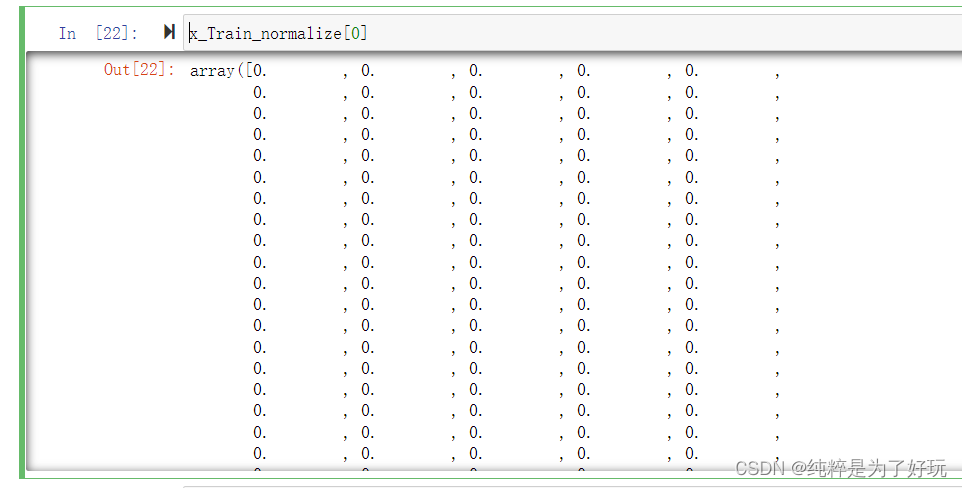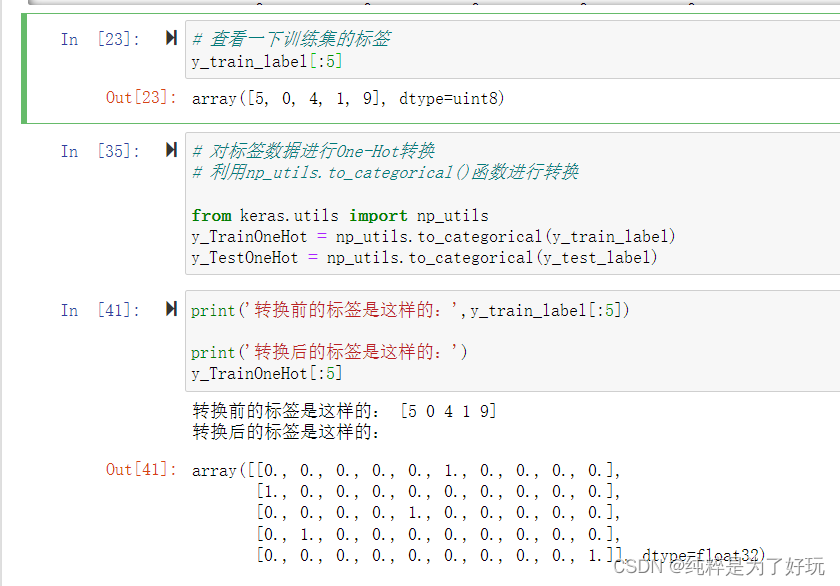## 5 建立模型

大家一定要注意： 输入层—神经网络的第一层。 它接收输入信号（值）并将其传递至下一层，但不对输入信号（值）执行任何运算。 它没有自己的权重值和偏置值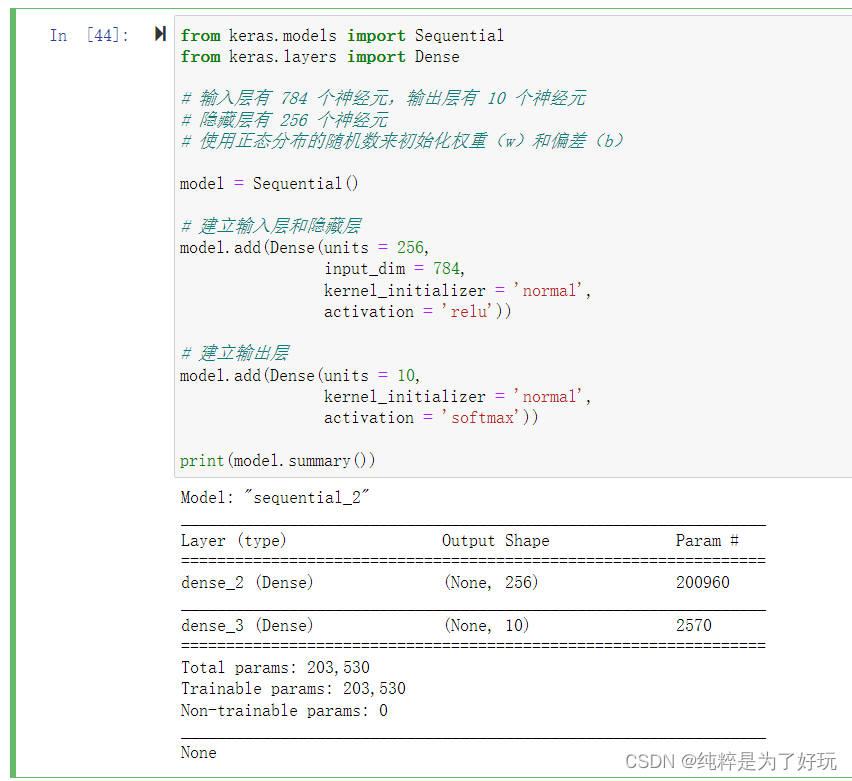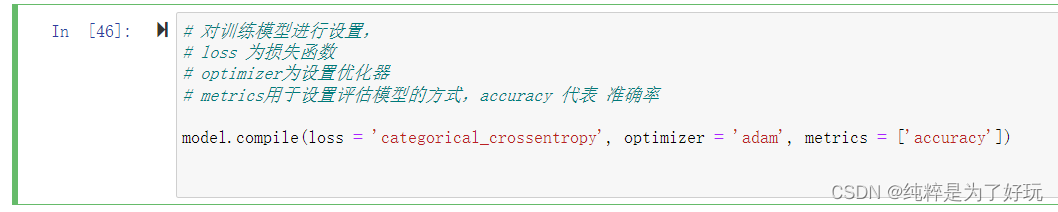## 6 训练模型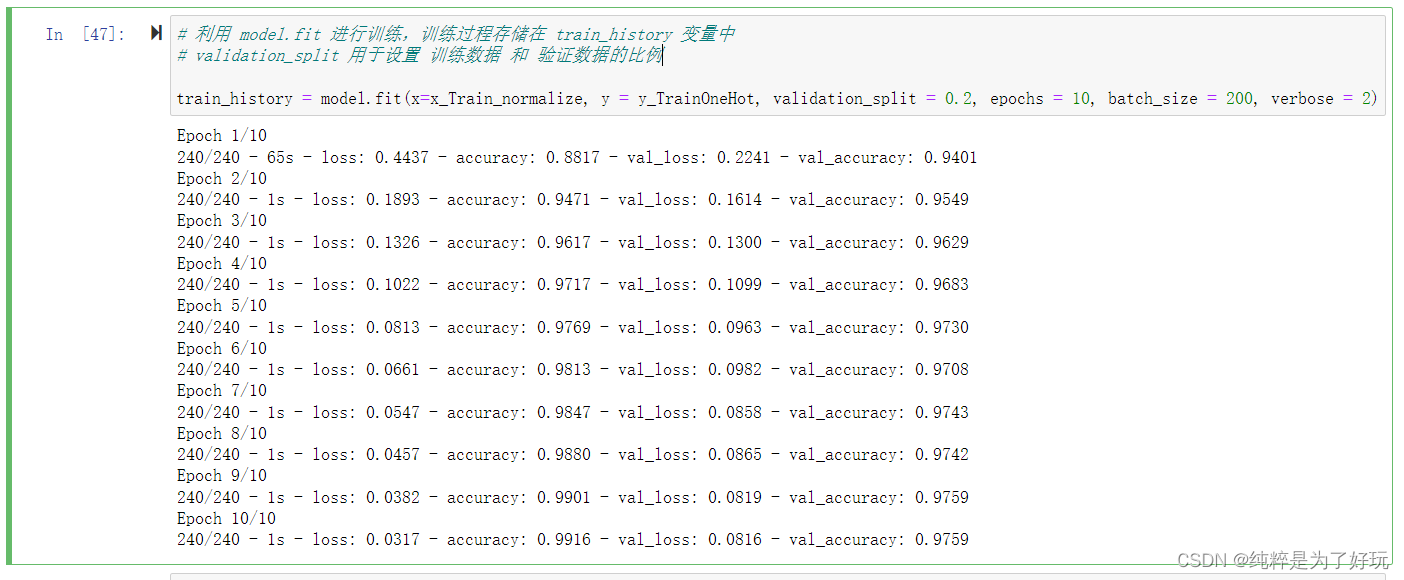## 7 评估模型准确率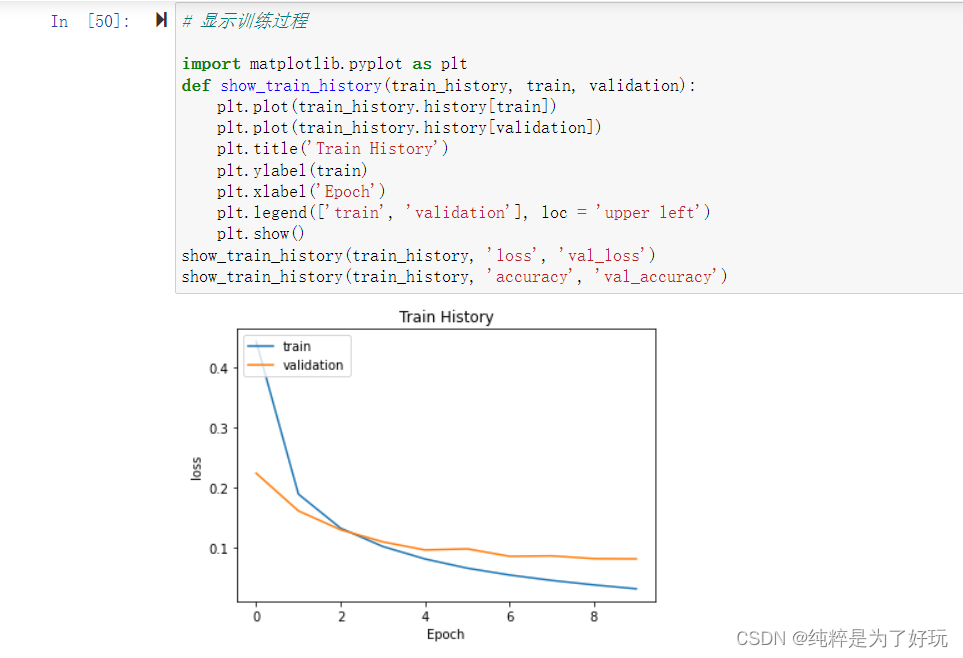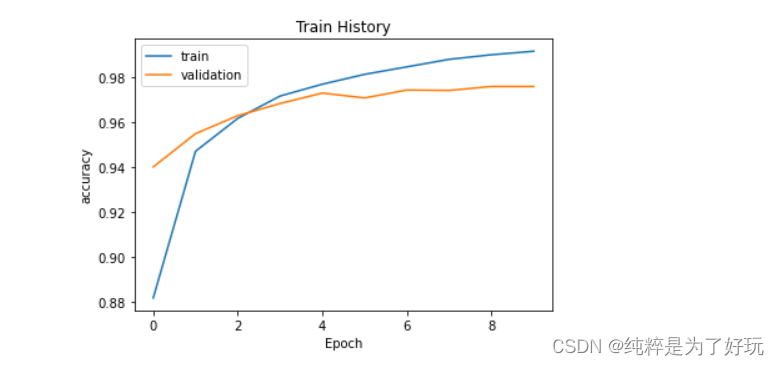## 8 进行预测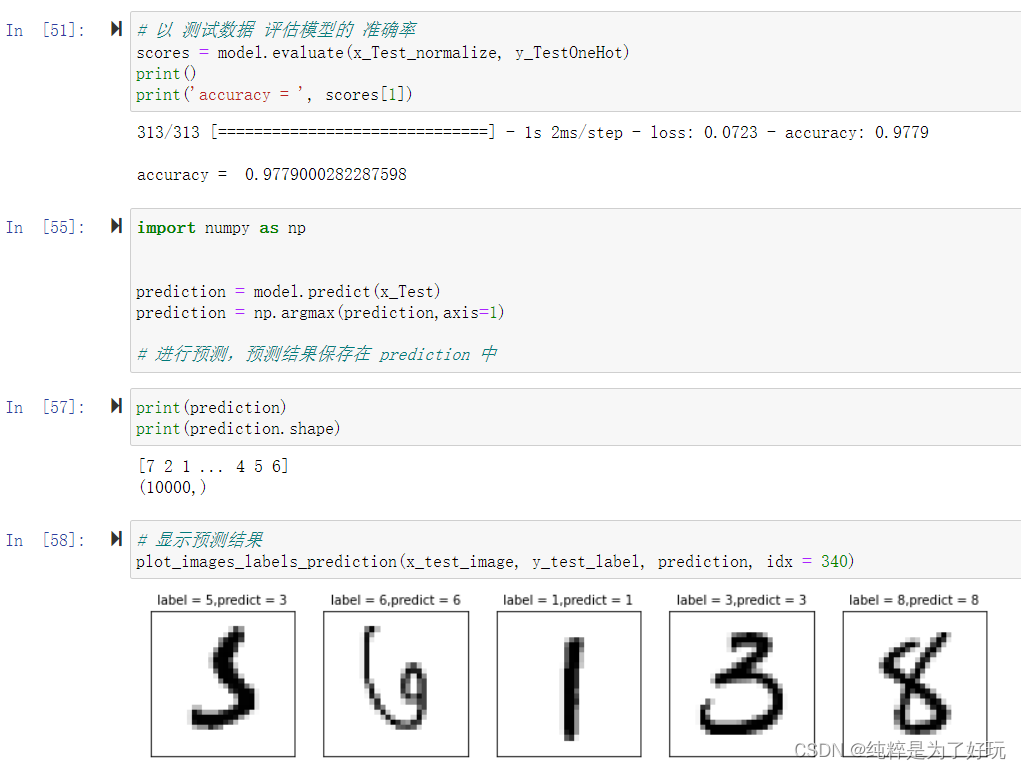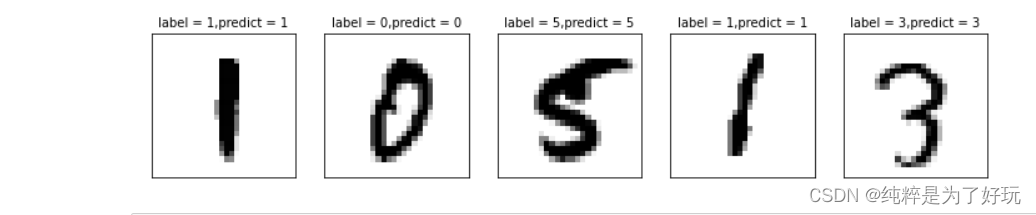展开全文keras python
• 配置完成后，在Jupyter Notebook中编写代码。首先引入PyTorch。 import torch import torchvision from torch.utils.data import DataLoader 2. 准备数据集 定义一些超参数： n_epochs = 3 #循环整个训练数据集...pytorch python
• 基于神经网络CNN的手写书字体识别代码，Jupyter运行。
• 1、MNIST手写识别问题 MNIST手写数字识别问题：输入黑白的手写阿拉伯数字，通过机器学习判断输入的是几。可以通过TensorFLow下载MNIST手写数据集，通过import引入MNIST数据集并进行读取，会自动从网上下载所需文件...逻辑回归模型
• 环境配置好了，就可以进行第一个程序了，实现mnist手写数字识别 我理解的原理就是通过将图片上的像素量化，并设置权重值来识别图片内容，具体的跑一个程序看看 开始导入数据 import tensorflow as tf from ...python
• 分享给大家供大家参考，具体如下：1、MNIST手写识别问题MNIST手写数字识别问题：输入黑白的手写阿拉伯数字，通过机器学习判断输入的是几。可以通过TensorFLow下载MNIST手写数据集，通过import引入MNIST数据集并进行...
• 下面是100个epochs的全部，识别的输出，其它lr初始值为0.01，而不是0.001。 Epoch 1/100 lr change to 0.01 235/235 [==============================] - 2s 7ms/step - loss: 0.1065 - acc: 0.9673 - val_loss: 0....神经网络 tensorflow
• Mnist数据集中的标签是介于0~9的数字Mnist中的标签是用独热编码(one-hot-vectors)表示的，一个one-hot向量除了某一位数字是1以外，其余维度的数组都是0，比如标签0用独热编码表示为([1, 0, 0, 0, 0, 0, 0, 0, 0, ...
• 深度学习100例 —— 卷积神经网络（CNN）实现mnist手写数字识别python
• MNIST手写数字识别 本节将学习机器学习的分类开发应用，即MNIST手写数字识别。对此，我们通过建立一个两层神经网络的模型来用于预测图片里面的数字。 MNIST数据集介绍 MNIST是一个非常有名的手写体数字识别数据...机器学习 tensorflow 神经网络
• Tensorflow Mnist手写数字识别学习1准备工作定义模型定义占位符定义神经网络和前向传播过程损失函数及优化器定义准确率超参数及参数的设置训练模型训练结果 准备工作 %matplotlib inline import matplotlib.pyplot ...Tensorflow mnist手写数字 人工智能 图像识别
• 本文利用Python语言，在Jupyter Notebook进行程序编写，通过神经网络学习算法对MNIST手写数据集进行数字识别，并且对学习率（learning rate）、节点数（nodes）进行分析，找出最佳的学习率和节点数，使得神经网络对...python 机器学习 神经网络
• TensorFlow是一个基于Python和基于数据流编程的机器学习框架,而MNIST是一个经典的计算机视觉数据集，本文是基于TensorFlow卷积神经网络与MNIST数据集设计手写数字识别算法。tensorflow MNIST 手写数字识别算法 python 机器学习
•神经网络 python
• 手写数字识别（图像，视频，实时摄像头）MNIST DATASET（手把手教程）:Jupyter+Tensorflow 本实验要用到 Anaconda+Jupyter+Tensorflow没有环境的看这：链接: Anaconda+Jupyter+Opencv+tensorflow安装.有任何问题评论...python tensorflow 图像识别
• 多层感知器MLP和卷积神经网络CNN识别手写数字Mnist，使用Jupyter Notebook 编写的 Python代码，含建模及测试，代码注释清晰，十分适合新手
• 周末根据Tariq Rashid大神的指导，没有使用tensorflow等框架，用python编写了一个三层神经网络，并应用再mnist手写识别上，经过多方面参数调优，识别率竟然达到了98%。 调优比较难，经验感觉特别宝贵，为避免时间...python 神经网络 机器学习
• 本文使用的CNN网络将MNIST数据的识别率提高到了99%。下面我们就开始进行实战。 导入包 import torch import torch.nn as nn import torch.nn.functional as F import torch.optim as optim from torchvision imp
• 基于mnist数据集的手写数字识别：包含mnist数据集+jupyter notebook代码+测试图片 不包含任何python框架，新手入门神经网络神器，调整超参数就会有意想不到的惊喜
• 今天小编就为大家分享一篇使用PyTorch实现MNIST手写识别代码，具有很好的参考价值，希望对大家有所帮助。一起跟随小编过来看看吧 文章目录实验环境MNIST数据集介绍导入包定义超参数数据集定义网络实例化网络定义...
• 本文详细介绍了如何构建LeNet-5神经网络用于手写数字识别。 文中大量的代码解释包含在代码行后的注释中，请注意查看。 下面的代码在谷歌云盘的colab上运行，也可以在jupyter notebook上运行...